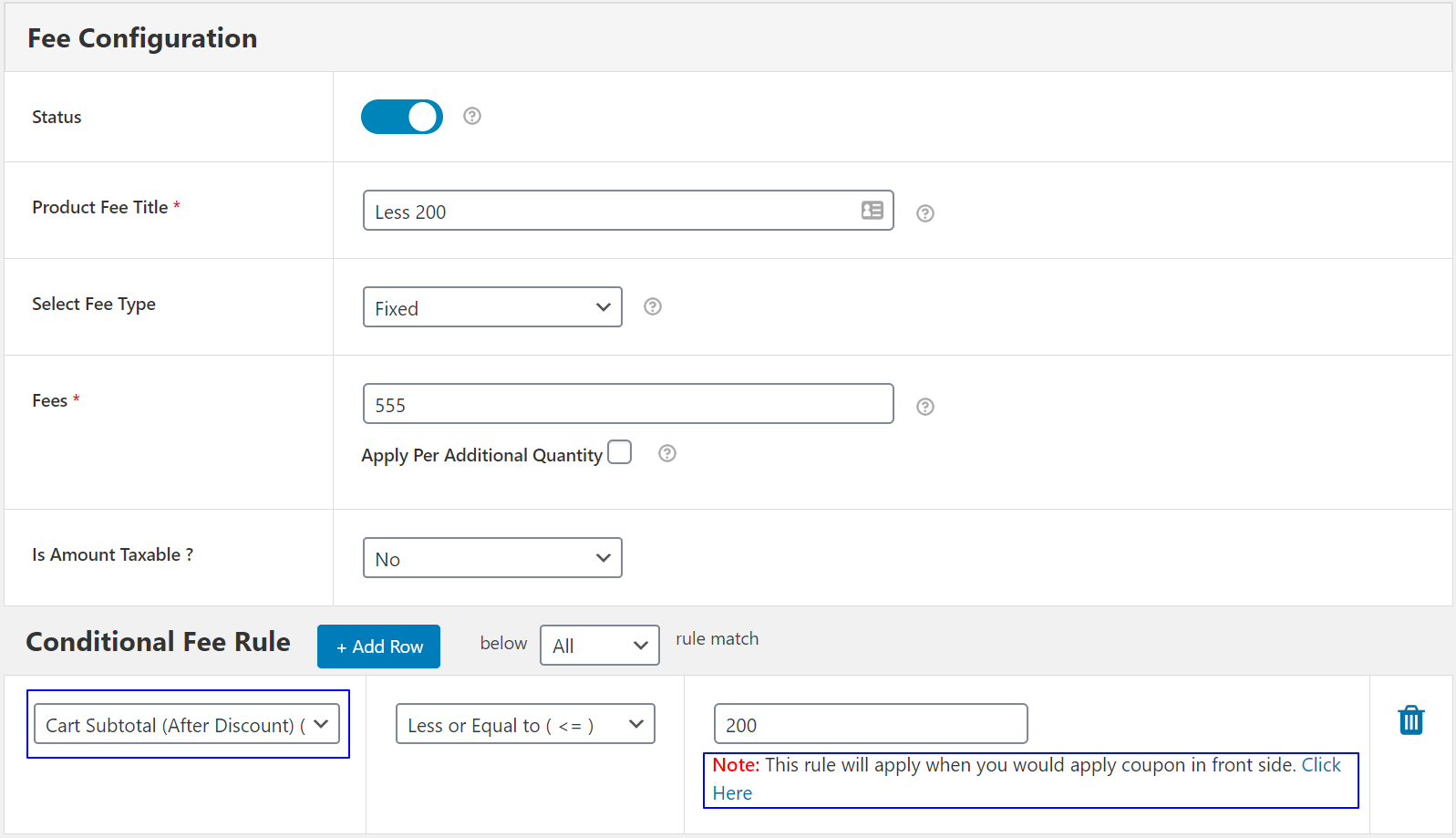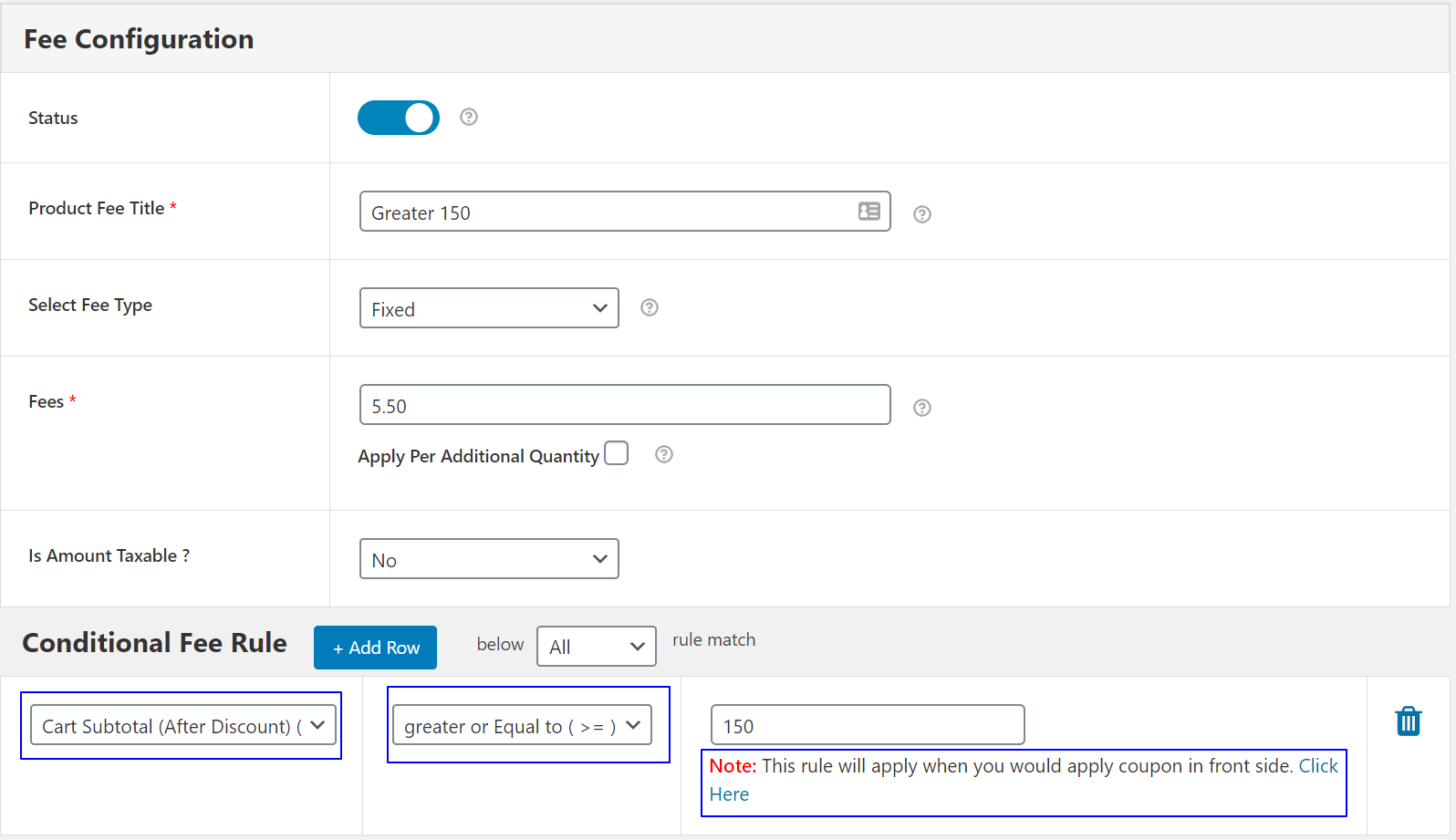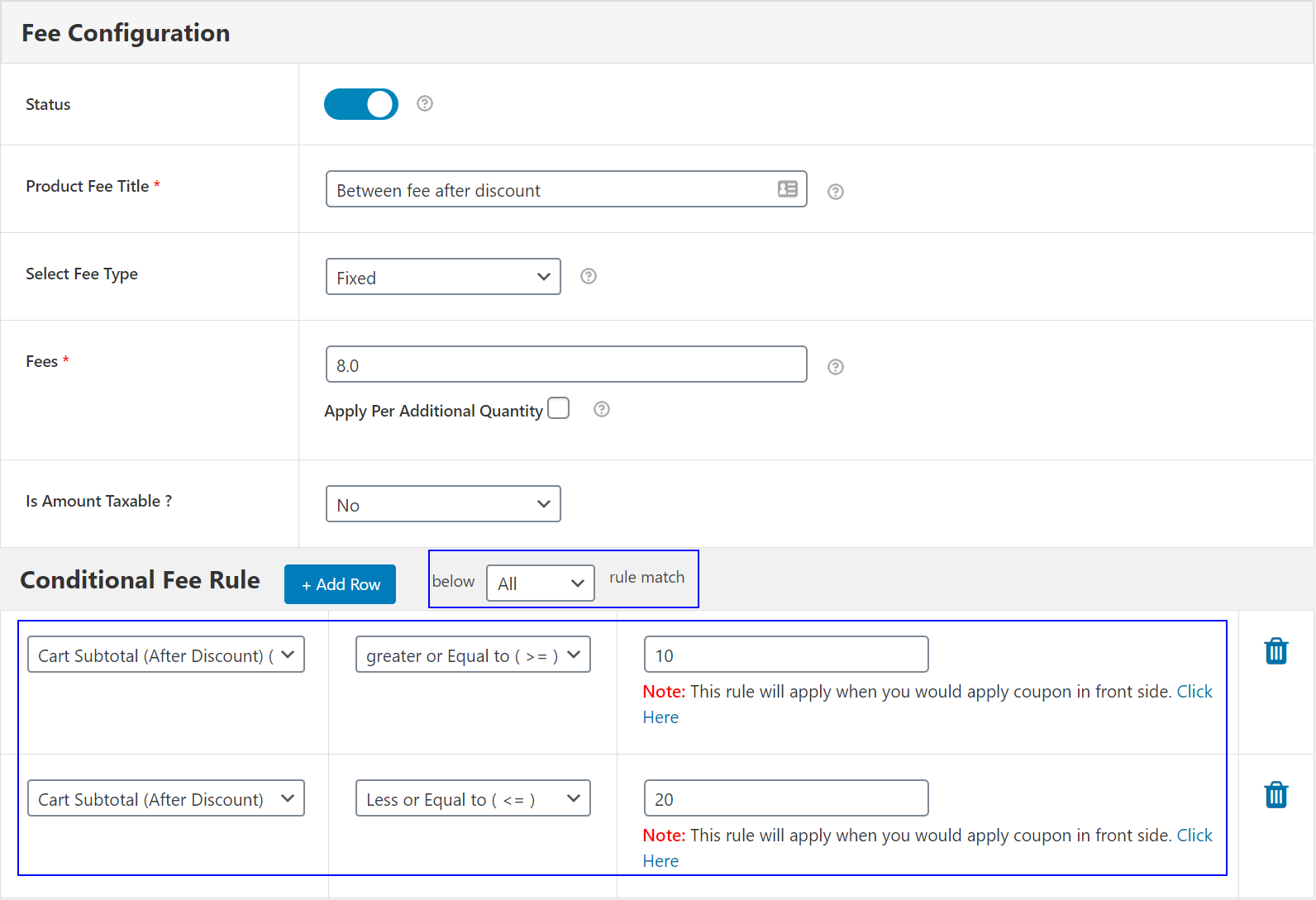# How to add fee based on After Discount rule

Once we need to add fees based on the cart subtotal after discount coupon applied then, we can use this After Discount rules.

List example: How to enable conditional fee on the basis of cart subtotal after discount code applied.
We have three instances here:

1 If the Cart Total is less than \$500 after discount, then only allow local fees.

2 If Cart Total is between \$501 – \$1000 after discount, then allow Medium fee.

3 If Cart Total is Above \$1000 after discount, then allow No fees.

Cart subtotal Conditions parameter as per below:

With Cart subtotal after the discount (order amount fee) rules, you can apply a different condition on Cart subtotal as per below.

• = Equal to
• > Greater than
• < Less than
• != Not equal to
• >= Greater than or equal to
• <= Less than or equal to

Note: Cart Subtotal amount = Order Amount after all the discount coupon applied. If no coupon applied then, this rule will not work.

1. If Cart subtotal after the discount  is LESS THAN

Here you can enter the maximum order amount to for which the fees should be visible.
Example: You can specify the order amount OR Cart subtotal is < 200. In this case, the fees will only be visible to the customer.
if the order amount OR Cart subtotal is less than and equal to <= 200. For orders more than 200, it’s will not be visible.

You can use this feature when you want to skip the fees charge when the order amount is higher than what you specified.2.  If Cart subtotal before the discount is  GREATER THAN

Here you can enter the minimum order amount after which the fees should be visible
Example: You can specify the order amount greater than >=50. In this case, the fees will only be visible to the customer.
if the order amount is greater than and equal to 50. For orders smaller than 50 it will not be visible.

You can use this feature when you want to skip fees charges when the order amount is less than what you specified.3. If Cart subtotal before the discount IS BETWEEN
Here you can create range wise fees. like 10 to 20.
Example: You can create range wise fees on order amount like \$10 to \$20 then charge \$24.
In this case, the fees will only be visible to the customer if the order amount is between \$10 to \$20 then it will visible fee charges \$24 on the cart.
If the order amount is not between a particular range then it will not visible.